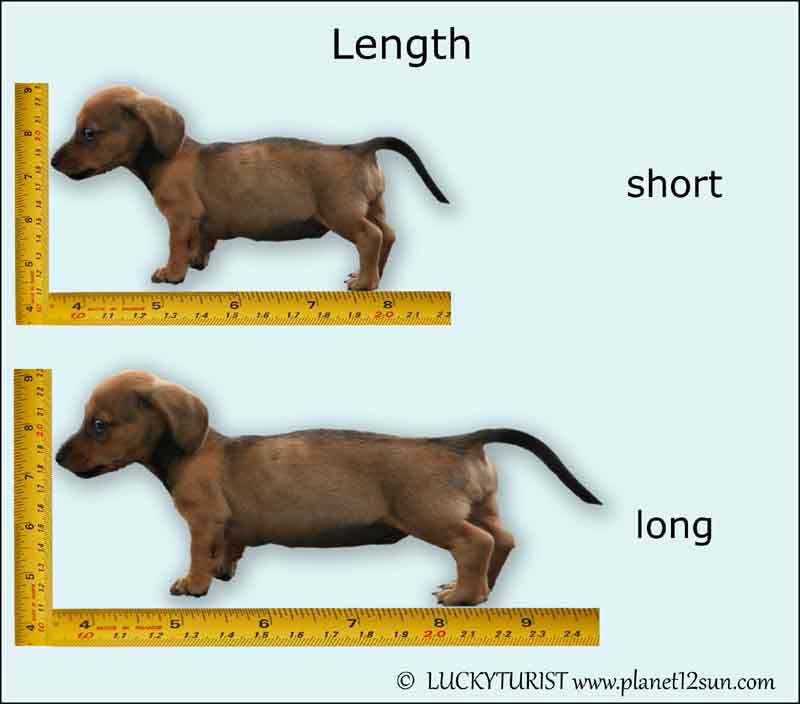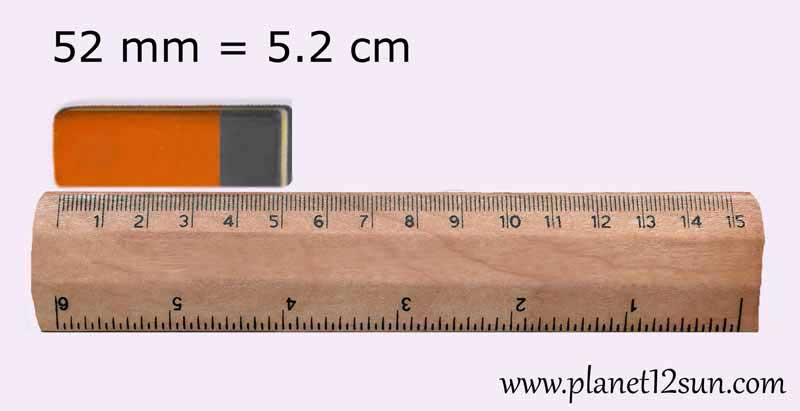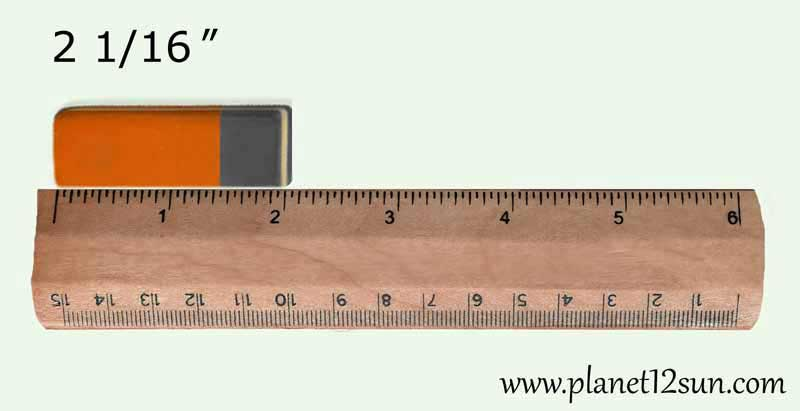## Length

The length of something is the distance between two ends of the thing. In the physical sciences and engineering, when one speaks of "units of length", the word "length" is synonymous with "distance". For two dimensional things, length is usually the longer side of measurement.
Short means of small length. Long means of much length. Short and long are opposites.

### Metric System

millimeter (mm), centimeter (cm), decimeter (dm), meter (m), kilometer (km)

1 cm = 10 mm
1 dm = 10 cm
1 m = 10 dm
1 m = 100 cm
1 km = 1000 m

Millimeters
Small objects are measured in millimeters (mm). It is one the most commonly used units of length which equals to 1/1000th of a meter (m).

There are many things that can be measured in millimeters: fingernails, buttons, pencil lead, wire diameters....

How to Measure Length in Millimeters
The simplest way to measure the length of an object in millimeters is to use a ruler with metric measurements. A metric ruler has larger lines with numbers corresponding to centimeters. There are also 10 fraction marks (or dashes) between two consecutive centimeter lines. Each of these smaller marks represents a millimeter, which is one-tenth of a centimeter (cm).

Place the zero mark of the ruler at the left end of an object.
Align the ruler with the object.
Read the last centimeter number on the ruler at the other end of the object being measured. (For example, we'll say it is 5 centimeters).
Count how many fraction marks the object you are measuring extends beyond the last whole number. (For example, the number of small dashes is 2).
Multiply centimeters by 10 and add the number of fraction marks to get the length in millimeters. In the example, the length is 5 times 10 plus 2, or 52 millimeters.Centimetres
Longer objects can be measured in centimetres.

There are many things that can be measured in centimetres: fingers, pencils, matches, feet, paper, tv screen...

How to Measure Length in Centimetres
Use a metric ruler.
Place the zero mark of the ruler at the left end of an object.
Align the ruler with the object.
Read the last centimeter number on the ruler at the other end of the object being measured. (For example, we'll say it is 5 centimeters).
Count how many fraction marks (mm) the object you are measuring extends beyond the last whole number. (For example, the number of small dashes is 2).
Divide the number of mm by 10 and add it to the centimeter number. In the example, the length is 2 divided by 10 plus 5, or 5.2 cm.

Meters
Long objects can be measured in meters.

There are many things that can be measured in meters: furniture, carpet, garden, height of a person (the average female height is about 1.6 meters), length of the track....

You can use a retractable tape measure or a traditional ribbon-style tape measure to measure length in meters.

Kilometers
The distance from one city to another or how far a plane travels are measured using kilometers. A kilometer is equal to 1,000 meters.

### US Standard Units (English Units, US Customary Units)

inches (in), feet (ft), yards (yd), miles (mi)

Small units of length are called inches. Plural: inches; abbreviation or symbol: "in" or ″ (a double prime).

There are many things that can be measured in inches: table, tv screen, paper, building materials...

When you have 12 inches together, it is known as a foot.

1 foot = 12 inches

The shortest way of writing the unit "foot" is by the abbreviation "ft" or by an apostrophe ( ' ).

One foot contains 12 inches which is equal to 30.48 centimetres. It is called a foot, because it was originally based on the length of a foot.When 3 feet are together, this is called a yard (abbreviation yd, plural sometimes yds).

1 yard = 3 feet

There are many things that can be measured in yards: backyard, lawn, football field...

When you put together 1,760 yards, you have a mile.

1 mile = 1,760 yards = 5,280 feet

Miles are long distances and are mostly used to measure the distance between places which are far away from each other. Most people refer to miles when they are driving, biking or jogging.

How to Measure in Inches
You'll need a tool that measures length in inches. The usual options would be a ruler, yardstick, or tape measure. Rulers are best for short distances. Yard sticks are better for slightly longer distances. Use a tape measure when you need to measure the distance around a curved object (it can bend).
Line up the “0” end with one side of the length to be measured.
Read the inch value. Locate the numbered value on the measuring tool that falls out immediately before the opposite end of the edge of the object being measured. This numbered value is the number of whole inches in length.
The shorter, unnumbered lines in between the numbered values are fractions of an inch. Each inch is broken down into 1/16 of an inch.
Count the unnumbered lines past the previous inch value.
Add the fraction to the inch value just counted onto the whole inch value you already measured. This should give you your final measurement. (Don't forget to simplify the fraction. Learn how to simplify fractions here.)